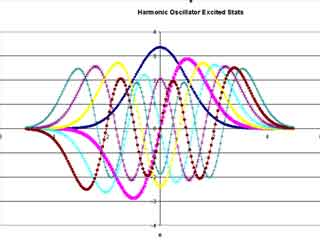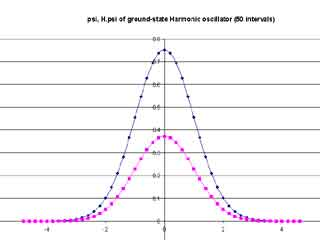# Wherein I Give and Receive Cryptic Messages

Someone sent me an SMS which I couldn’t really understand – text as follows:

Hi min, gud evng kmsta na? Na masyal ka kanina?

I ignored it, but the next day another one came, which I am unable to reproduce as I deleted it in annoyance. So I replied that the guy had the wrong number and that I was no “min”. He (I assume it’s a he) replied with a message that had the words “what” and “name” in there somewhere, so I assumed he was requesting my identity.

I gave it to him and never got a reply.

A search on Yahoo! revealed (I hope) the language to be phillipino.

Sometimes people ask me what I study. A course in which the average class size is smaller than 10 would be strange enough to warrant some interest, I guess. I’ll describe a lab I just finished.

Question 1: Plot the first six excited states (normalized) of the wavefunction of a (quantum) Harmonic Oscillator by using Hermitian operators as the bases.

If you’ve taken any quantum (or classical mechanical) physics module you should understand what the above statement means. Otherwise it’s really just some squiggly lines – the trick lies in the source code, which for my labs is usually less than a hundred lines, much much less than the Computing student’s.

Thing is, our main job is to find the constants that get you the results you want and to get the equations to plot in the first place. Short code means more pen-and-paper equation-writing (which I hate). And, of course, to find the bloody error involved when you perform numerical quadrature instead of analytical integration. In the above case, accuracies of 0.001% could be obtained after about 5000 intervals were used in the case of the ground state.Question 2: Use the Central Difference Therorem to test the Schrodinger equation for the 0th excited state of a Harmonic Oscillator and confirm the eigenenergy of 0.5(depending on state variables).

Okay, this one was easy too, although a little difficult to explain – basically the Schrodinger equation (H.psi = E0.psi?) allows one to predict wavefunctions that can correspond to specific eigenenergies (quantum states can only exist at certain energies, thus the quantum honorific) and from Question 1 we managed to find the wavefunction, so here we use the Central Difference Method (a simple algorithm to calculate differentials) to test out the results.And the results are exactly what we want, showing a perfect 0.5 correspondence between the Hamiltonian and the wavefunction.

Whee.

Understanding the question (copied hastily during lectures in poor handwriting with little-to-no comprehension of the subject matter) takes me about two to three hours. Writing the program takes me something like a half hour tops. Debugging and adjusting constants takes hours, in this case two days. I had forgotten to normalize wavefunctions at one point. My gaussian curves looked funny at another because I had neglected a ratio of 0.5 in the exponential.

All this for a couple of squiggly lines.

So really, is it any wonder that I don’t want to get into a job to do with my major?